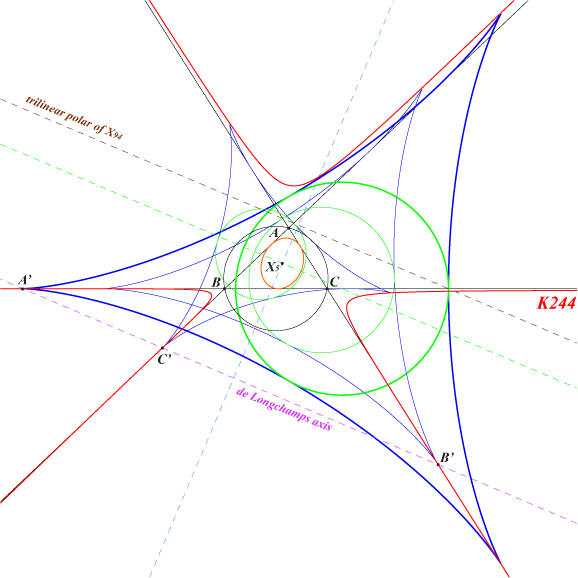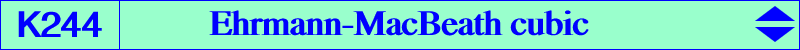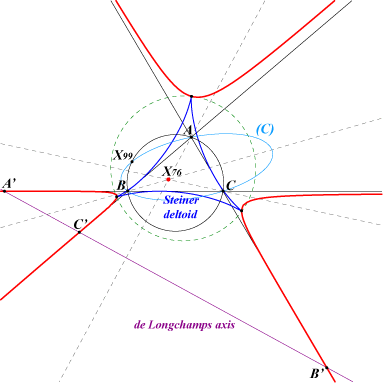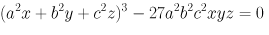X(76), X(764), X(23097), X(23098), X(23099), X(23100), X(23101), X(23102), X(23103), X(23104), X(23105), X(23106), X(23107), X(23108), X(23109), X(23110) X(58252) → X(58257) extraversions of X(764) imaginary foci of the MacBeath inconic cusps of the Steiner deltoid and, more generally, of any deltoid inscribed in ABC A', B', C' : feet of the de Longchamps axis (trilinear polar of X(76), isotomic transform of the circumcircle), these are flexes on the cubic.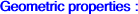K244 is the trilinear cube of the line at infinity. The barycentric cube of the line at infinity is K656. The locus of the trilinear poles of the lines tangent to a deltoid touching the sidelines of ABC is an acnodal cubic with node G, member of the pencil generated by the three medians and the Simson cubic K010. Since the pencil above is selfisotomic, these cubics are the duals of the deltoids (the Simson cubic being the dual of the Steiner deltoid). The locus of the cusps of the deltoids touching the sidelines is the Ehrmann-MacBeath cubic K244. See below for a construction of these deltoids. The locus of the contacts of these deltoids with their incircles is the Steiner-MacBeath cubic K583. K244 is an acnodal cubic with node X(76), the isotomic conjugate of the symmedian point K. The MacBeath conic is the envelope of the incircles of all the deltoids inscribed in ABC (there are infinitely many such deltoids, of which Steiner's is the smallest). The locus of their centers is the perpendicular bisector of OH. K244 touches the sidelines of ABC at their common points A', B', C' with the trilinear polar of X(76) and these common points are flexes. (Jean-Pierre Ehrmann, Hyacinthos #9941). The harmonic polars of A', B', C' are the cevians of X(76). The nodal tangents are parallel to the asymptotes of (C), the circum-ellipse whose perspector is X(3314), the intersection of the lines X(2)X(6) and X(76)X(115). The axes of (C) are parallel to the asymptotes of the Kiepert hyperbola which turns out to be the polar conic of X(523) in the cubic. K244 is the envelope of the trilinear polars of points on the inscribed conic with perspector X(76), center X(141). See a generalization and a construction in the page containing the related cubic K656. See K1065 for other properties and a related transformation. See also "Hypocycloïdes et épicycloïdes" by J. Lemaire, librairie Albert Blanchard, Paris, 1967.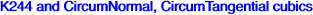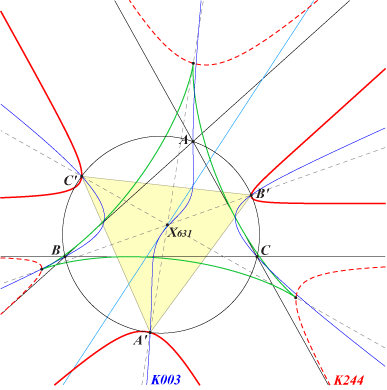Let h be the homothety with center X(631) and ratio -2/3. h maps the circle with center X(5), radius 3R/2 to the circumcircle and K244 to a cubic passing through the vertices of the circumnormal triangle, the images of the cusps.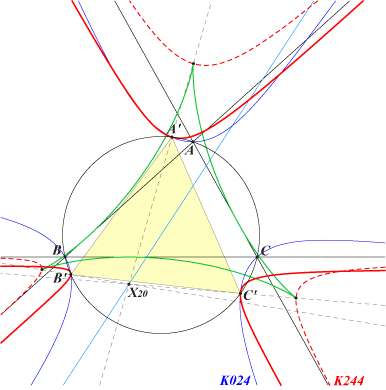Let h' be the homothety with center X(20) and ratio 2/3. h' maps the circle with center X(5), radius 3R/2 to the circumcircle and K244 to a cubic passing through the vertices of the circumtangential triangle, the images of the cusps.Conversely, • the homothety with center X(631) and ratio -3/2 maps any circumnormal cubic to a cubic through the cusps of the Steiner deltoid, • the homothety with center X(20) and ratio 3/2 maps any circumtangential cubic to a cubic through the cusps of the Steiner deltoid. See Table 25 for examples of such cubics.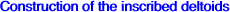Let t ≠ 0 be any angle in [-π/2 ; π/2] and let M be a variable point on the circumcircle of ABC. The parallel at M to BC is rotated by t about M giving a line denoted by La meeting BC at Ma. Two other lines Lb, Lc and two other points Mb, Mc are defined similarly. Ma, Mb, Mc are collinear on a line L which is sometimes called the generalized Simson line of M. When M traverses the circumcircle, the envelope of L is a deltoid inscribed in ABC. Obviously, when t = ± π/2, we obtain the usual Simson lines and then the Steiner deltoid. Note that two opposite values for t give two deltoids with centers symmetric about X(5) and whose incircles have the same radius which is r = R / (2 |sin t|). In particular, when t = ± π/6, the radii of the incircles is R. The incircles of all these deltoids are the circumcircles of the triangles inscribed in ABC and similar either to ABC or to the orthic triangle.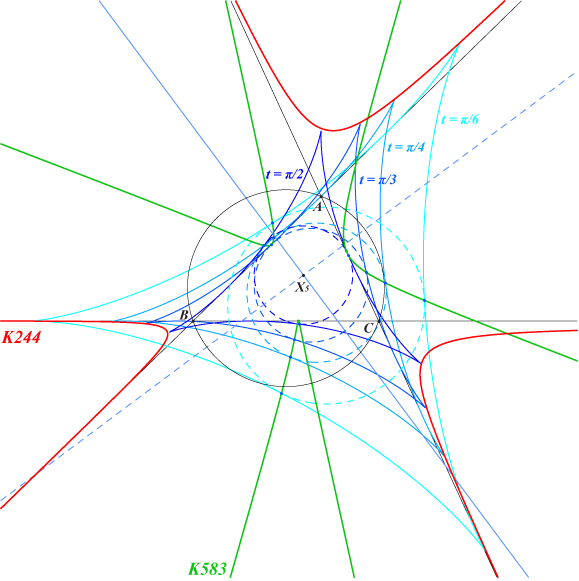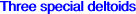There are three deltoids having a cusp at one of the points A', B', C'. One of their axes of symmetry is a sideline of ABC.